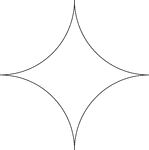### Reflected Arcs Of A Circle

A design created by dividing a circle into 4 equal arcs and reflecting each arc toward the center of…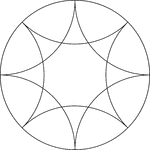### Reflected Arcs Of 2 Circles In A Circle

A design created by dividing a circle into 4 equal arcs and creating a reflection of each arc toward…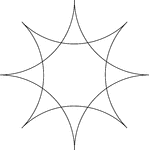### Reflected Arcs Of 2 Circles

A design created by dividing a circle into 4 equal arcs and reflecting each arc toward the center of…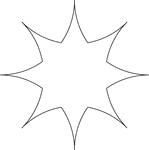### Reflected Arcs Of 2 Circles

A design created by dividing a circle into 4 equal arcs and reflecting each arc toward the center of…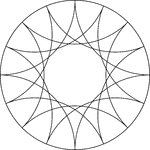### Reflected Arcs Of 4 Circles In A Circle

A design created by dividing a circle into 4 equal arcs and creating a reflection of each arc toward…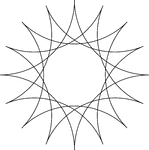### Reflected Arcs Of 4 Circles In A Circle

A design created by dividing a circle into 4 equal arcs and creating a reflection of each arc toward…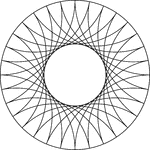### Reflected Arcs Of 8 Circles In A Circle

A design created by dividing a circle into 4 equal arcs and creating a reflection of each arc toward…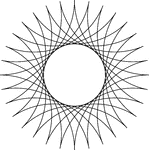### Reflected Arcs Of 8 Circles

A design created by dividing a circle into 4 equal arcs and creating a reflection of each arc toward…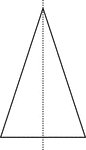### Vertical Line of Symmetry, Isosceles Triangle With

Isosceles Triangle with a dotted vertical line that is a line of symmetry.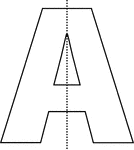### Vertical Line of Symmetry, Letter A With

Letter A with a dotted vertical line that is a line of symmetry.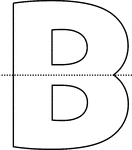### Horizontal Line of Symmetry, Letter B With

Letter B with a dotted horizontal line that is a line of symmetry.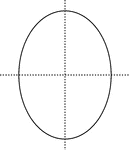### Lines of Symmetry, Oval With

Oval with dotted vertical and horizontal lines that are lines of symmetry.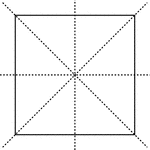### Lines of Symmetry, Square With

Square with dotted vertical, horizontal, and diagonal lines that are lines of symmetry.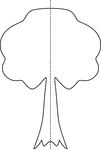### Line of Symmetry, Tree With

Tree with dotted vertical line that shows symmetry.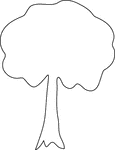### Symmetry, Tree With No

Tree that has no symmetry.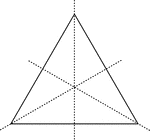### Lines of Symmetry, Triangle With

Square with dotted lines that are lines of symmetry.